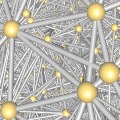CGAL 5.3.1 - 3D Triangulations
3D Triangulations ReferenceClément Jamin, Sylvain Pion and Monique Teillaud
This package allows to build and handle triangulations for point sets in three dimensions. Any CGAL triangulation covers the convex hull of its vertices. Triangulations are build incrementally and can be modified by insertion, displacements or removal of vertices. They offer point location facilities. The package provides plain triangulation (whose faces depends on the insertion order of the vertices) and Delaunay triangulations. Regular triangulations are also provided for sets of weighted points. Delaunay and regular triangulations offer nearest neighbor queries and primitives to build the dual Voronoi and power diagrams. Optionally, the main Delaunay and regular triangulation algorithms (insert, remove) support multi-core shared-memory architectures to take advantage of available parallelism.
Introduced in: CGAL 2.1
Depends on: 3D Triangulation Data Structure
BibTeX: cgal:pt-t3-21b
Windows Demo: 3D Triangulations
Common Demo Dlls: dlls

A three-dimensional triangulation is a three-dimensional simplicial complex, pure connected and without singularities . Its cells (3-faces) are such that two cells either do not intersect or share a common facet (2-face), edge (1-face) or vertex (0-face).

The basic 3D-triangulation class of CGAL is primarily designed to represent the triangulations of a set of points $$A$$ in $$\mathbb{R}^3$$. It can be viewed as a partition of the convex hull of $$A$$ into tetrahedra whose vertices are the points of $$A$$. Together with the unbounded cell having the convex hull boundary as its frontier, the triangulation forms a partition of $$\mathbb{R}^3$$.

In order to deal only with tetrahedra, which is convenient for many applications, the unbounded cell can be subdivided into tetrahedra by considering that each convex hull facet is incident to an infinite cell having as fourth vertex an auxiliary vertex called the infinite vertex. In that way, each facet is incident to exactly two cells and special cases at the boundary of the convex hull are simple to deal with.

A triangulation is a collection of vertices and cells that are linked together through incidence and adjacency relations. Each cell gives access to its four incident vertices and to its four adjacent cells. Each vertex gives access to one of its incident cells.

The four vertices of a cell are indexed with 0, 1, 2 and 3 in positive orientation, the positive orientation being defined by the orientation of the underlying Euclidean space $$\mathbb{R}^3$$. The neighbors of a cell are also indexed with 0, 1, 2, 3 in such a way that the neighbor indexed by i is opposite to the vertex with the same index. See Figure 45.1.

Modules

Concepts

Triangulation Classes

Traits Classes

Vertex and Cell Classes

Draw a Triangulation 3

I/O for a Triangulation 3

Functions

template<class Triangulation , class TriangleMesh >
boost::graph_trait< FG >::vertex_descriptor CGAL::link_to_face_graph (const Triangulation &t, typename Triangulation::Vertex_handle vh, TriangleMesh &tm, bool no_infinite_faces=true)
fills the face graph tm with the link of triangulation vertex vh. More...

Function Documentation

template<class Triangulation , class TriangleMesh >
 boost::graph_trait::vertex_descriptor CGAL::link_to_face_graph ( const Triangulation & t, typename Triangulation::Vertex_handle vh, TriangleMesh & tm, bool no_infinite_faces = true )